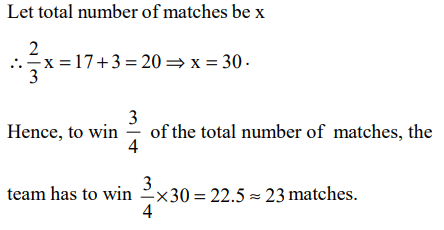## Algebra Questions and Answers Part-1

1. If a + b + c = 0, where $a\neq b \neq c$  , then, $\frac{a^{2}}{2a^{2}+bc}+\frac{b^{2}}{2b^{2}+ac}+\frac{c^{2}}{2c^{2}+ab}$      is equal to
a) zero
b) 1
c) -1
d) abc

Explanation:2. If one root of $x^{2}+px+12=0$    is 4, while the equation $x^{2}+px+q=0$    has equal roots, then the value of q is
a) 49/4
b) 4/49
c) 4
d) 1/4

Explanation: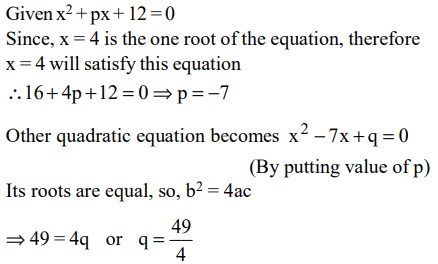3. Nineteen years from now Jackson will be 3 times as old as Joseph is now. Joseph is three years younger than Jackson.
I. Johnson’s age now
II. Joseph’s age now
a) I > II
b) I < II
c) I = II
d) Nothing can be said

Explanation: Let present age of Jackson be x years and present age of Joseph be y years; then present age of Joseph = x – 3
After 19 years Jackson’s age = (x + 19) years and
Joseph’s age = (y + 19) years.
According to the question:
x + 19 = 3(y + 19)
or x – 3y = 38
So only from above equation x and y cannot be found.

4. What is the value of m which satisfies $3m^{2}-21m+30$    < 0 ?
a) m < 2 or m > 5
b) m > 2
c) 2 < m < 5
d) m < 5

Explanation: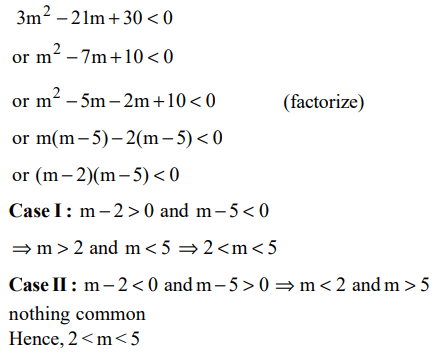5. The value of $\frac{55^{3}+45^{3}}{55^{2} - 55\times 45+45^{2}}$   is
a) 100
b) 105
c) 125
d) 75

Explanation:6. One root of $x^{2}+kx-8=0$    is square of the other. Then the value of k is
a) 2
b) 8
c) -8
d) -2

Explanation: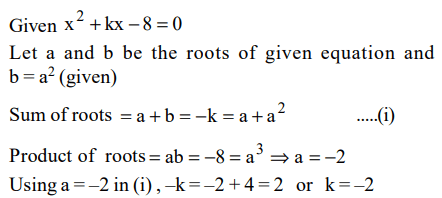7. Once I had been to the post-office to buy stamps of five rupees, two rupees and one rupee. I paid the clerk Rs 20, and since he did not have change, he gave me three more stamps of one rupee. If the number of stamps of each type that I had ordered initially was more than one, what was the total number of stamps that I bought?
a) 10
b) 9
c) 12
d) 8

Explanation: The number of stamps that were initially bought were more than one of each type. Hence, the total number of stamps
= 2(5 rupee) + 2(2 rupee) + 3(1 rupee) + 3(1 rupee)
= 10

8. Given the quadratic equation $x^{2}-\left(A-3\right)x-\left(A-7\right)$    , for what value of A will the sum of the squares of the roots be zero?
a) -2
b) 3
c) 6
d) None of these

Explanation: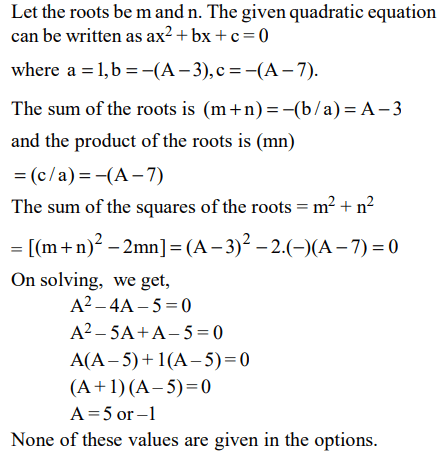9. Which of the following values of x do not satisfy the inequality $\left(x^{2}-3x+2>0\right)$    at all?
a) $1\leq x\leq 2$
b) $-1\geq x\geq -2$
c) $0\leq x\leq 2$
d) $0\geq x\geq -2$